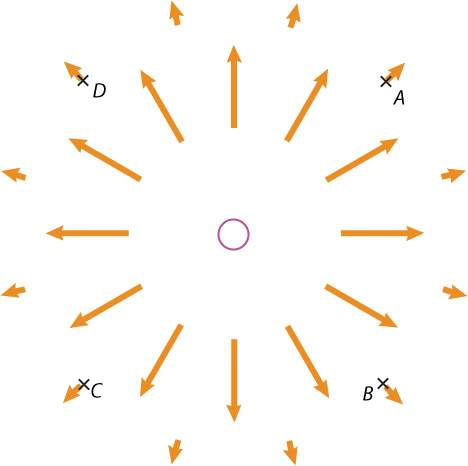# Electric Fields and source charge

## Homework StatementThe source charge (the magenta circle at the origin) is a positive charge. A particle whose charge is -6e-09 C is placed at location D.

1. The electric field at location D has the value < -8000, 8000, 0 > N/C. What is the unit vector in the direction of E at this location?

2. What is the electric force on the -6e-09 C charge?

3. What is the unit vector in the direction of this electric force?

E = kq/r^2

## The Attempt at a Solution

1. E = sqrt ( -8000^2 + 8000^2 + 0^2) = 11300
tan^-1(-8000/8000) = 135 degrees

11300=(8.99E9)(6E-9) / r^2
r=0.07 m
r(x)=0.07cos(135) = -0.05 m
r(y)=0.07sin(135) = 0.05 m

This is wrong. Can anyone show me how to do it? I assume you can't do #2 without knowing the value of #1, and you can't do #3 without knowing the value of #2? What exactly is question #2 and #3 asking?

cepheid
Staff Emeritus